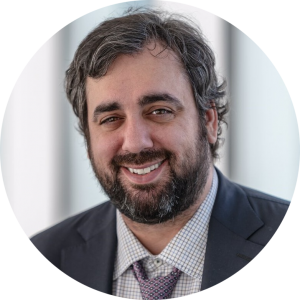# Laboratory for Quantum Information Processing (LQIP)Jacob Biamonte’s research focuses broadly on the theory and implementation of modern quantum algorithms and employs and develops various mathematical techniques, particularly group-algebraic techniques, tensor networks and the formal theory of computation and information. Biamonte is best known for several results: 1. A 2019 proof that variational quantum computation can be used as a computationally universal model of quantum computation [PRA (Letter), (2021)]. 2. A definition given in 2016 of a spectral graph function which provably satisfies both (i) the definition of an entropy and (ii) subadditivity [with Domenico in PRX 6, 041062 (2016)]. 3. A 2015 proof that #P-hard counting problems (and hence 2, 3-SAT decision problems) can be solved efficiently when their tensor network expression has at most O(log c) COPY-tensors and polynomial bounded fan-out [with Turner and Morton in J. Stat. Phys. 160, 1389 (2015)]. 4. A 2008 proof that the two-body model Hamiltonian with tunable XX, ZZ terms is (i) computationally universal for adiabatic quantum computation and (ii) admits a QMA-complete ground state energy decision problem [with Love in PRA 78, 012352 (2008)] Biamonte is further credited for pioneering work developing quantum algorithms for electronic structure calculations [Molecular Physics 109 (5), (2011)] and more recently for work uniting quantum information processing with machine learning. Biamonte has further provided theoretical support to enable milestone quantum information processing experimental demonstrations. The list includes the first quantum algorithmic demonstration of quantum chemistry [Nature Chemistry 2, 106 (2009)] (linear optics), the first experimental implementation of optimal control [Nature Communications 5, 3371 (2014)] (creating a quantum random access memory using NV-centers in diamond) as well as the first demonstration of neural network quantum state tomography on actual experimental data [npj Quantum Information 6:20 (2020)] (linear optics). Current projects include:  The theory and implementation of variational quantum algorithms (two projects) The mathematics of quantum information processing (one project)